# Fact families worksheet## Fact Families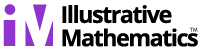Alignments to Content Standards:1.OA.B

Student View

#### Materials

• Fact Family work sheets

• Blank addition and subtraction frames (2 of each)

• Numbers cards in fact-family sets

#### Actions

As a whole class, the teacher presents students with 3 numbers on cards, such as 5, 8, and 3. The teacher asks the students to find all the ways the numbers can be put together in addition or subtraction sentences. The teacher will provide a set of frames, preferably on sentence strips in a pocket chart:

____ + ____ = ____

____ + ____ = ____

____ - ____ = ____

____ - ____ = ____

As the students determine the first equation, the teacher places the corresponding numbers in the pockets of the chart to represent it. Then the teacher points to the second addition sentence frame and asks,

Is there another addition equation we can write using these three numbers?

Once the students name the equation, the teacher then takes out another set of the same number cards and place them in the appropriate spots in the second equation.

The teacher then points out that the next equation is subtraction and asks the students to find a subtraction equation using the same three numbers. Both subtraction equations need to be determined, with the corresponding numbers placed in the pocket chart. When completed, the frames should look like this:

5 + 3 = 8

3 + 5 = 8

8 - 5 = 3

8 - 3 = 5

The students can chant in unison the 4 equations so they can both see and say them. Particularly at the beginning of first grade some students may confuse the +, -, and = symbols.

Then, in partners, students are given sets of 3 related numbers (see examples on the worksheet) and asked to find two addition and two subtraction equations with the same three numbers.

Once students are familiar with the objective, they are given the first worksheet. This practice can be given to individuals or partners to complete, or can be completed by the entire class together using a document projector with students coming up to write their equations.

### IM Commentary

The purpose of this task is for students to identify and write sets of related addition and subtraction equations; these are often known as "fact families" because the equations are related by the same underlying relationship between the numbers. This task reinforces the commutative property of addition and using the relationship between addition and subtraction. It is best given after the students have had quite a bit of experience adding and subtracting within 10. Note that there are actually 8 equations that can be written for every fact family; the attached worksheet has the 4 equation and 8 equation variants.

Creating the two addition equations will be easier for most children. When observing the subtraction equations the students create, one can determine their depth of understanding by noting whether or not the students use the minuend to begin the equation or the subtrahend. If some students are confused, cubes or manipulatives can be provided to model the equations. For a related task that employs pictures and encourages students to write eight related equations, see 1.OA Fact Families with Pictures.

To guide students who are experiencing confusion, or as an extension for those who show facility with the concept, arrows can be drawn connecting the same numbers in different equations. Alternatively, the same numbers can each be circled in a particular color (i.e. all 5’s circled in blue, 3’s circled in red, 8’s circled in green). This will indicate how each equation uses the same three numbers.

As an extension, students can create their own fact family numbers and corresponding equations, using the second worksheet without prepared numbers. A further extension to challenge advanced students would be to give students two numbers (i.e. 7 and 4) and ask them to find a third number that will form a fact family; here the student might add either 3 or 11. The student should then write the corresponding equations.

The term "fact family" is used here to talk about the special relationship between these addition and subtraction facts, similar to the idea that people in a family have a special relationship to each other because of deep connections they share. This metaphor was chosen because it is one that connects with students' lives. Math vocabulary such as addend, sum, minuend, subtrahend, and difference can be introduced in this task, but their use is not one of the primary objectives.

### Attached Resources

• triangle worksheet
• ### Solution### Fact Families

#### Materials

• Fact Family work sheets

• Blank addition and subtraction frames (2 of each)

• Numbers cards in fact-family sets

#### Actions

As a whole class, the teacher presents students with 3 numbers on cards, such as 5, 8, and 3. The teacher asks the students to find all the ways the numbers can be put together in addition or subtraction sentences. The teacher will provide a set of frames, preferably on sentence strips in a pocket chart:

____ + ____ = ____

____ + ____ = ____

____ - ____ = ____

____ - ____ = ____

As the students determine the first equation, the teacher places the corresponding numbers in the pockets of the chart to represent it. Then the teacher points to the second addition sentence frame and asks,

Is there another addition equation we can write using these three numbers?

Once the students name the equation, the teacher then takes out another set of the same number cards and place them in the appropriate spots in the second equation.

The teacher then points out that the next equation is subtraction and asks the students to find a subtraction equation using the same three numbers. Both subtraction equations need to be determined, with the corresponding numbers placed in the pocket chart. When completed, the frames should look like this:

5 + 3 = 8

3 + 5 = 8

8 - 5 = 3

8 - 3 = 5

The students can chant in unison the 4 equations so they can both see and say them. Particularly at the beginning of first grade some students may confuse the +, -, and = symbols.

Then, in partners, students are given sets of 3 related numbers (see examples on the worksheet) and asked to find two addition and two subtraction equations with the same three numbers.

Once students are familiar with the objective, they are given the first worksheet. This practice can be given to individuals or partners to complete, or can be completed by the entire class together using a document projector with students coming up to write their equations.

##Fact Family Worksheets### Fact Family Worksheets For Practice

These Fact Family Worksheets are perfect for practicing how to build the fact family sets for the given numbers for addition and subtraction as well as multiplication and division.

These Fact Family Worksheets are a great resource for children in Kindergarten, 1st Grade, and 2nd Grade.

### Quick Link for All Fact Family Worksheets

Click the image to be taken to that Fact Family Worksheet.

### Detailed Description for All Fact Family Worksheets

Addition and Subtraction Fact Family Worksheets
These Fact Family Worksheets are great for testing children in their ability to build fact families for addition and subtraction. You may select from the numbers 1 though 12 to be used in the problems. These Fact Family Worksheets will generate six problems per page.

Multiplication and Division Fact Family Worksheets
These Fact Family Worksheets are great for testing children in their ability to build fact families for Multiplication and Division. You may select from the numbers 2 though 11 to be used in the problems. These Fact Family Worksheets will generate six problems per page.

Adding and Subtracting Fact Family Problems
These Fact Family Worksheets allows you to pick the two addends to be used in the addition and subtraction fact families problems. These Fact Family Worksheets will generate six problems per page.Sours: https://www.math-aids.com/Fact_Family/

Comment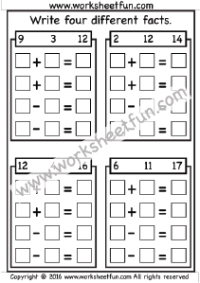Fact Family – Write four different facts – 6 Worksheets

Comment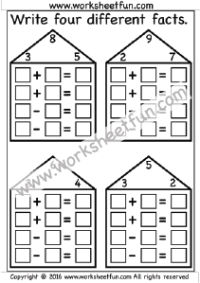Fact Family Houses – 5 Worksheets

Comment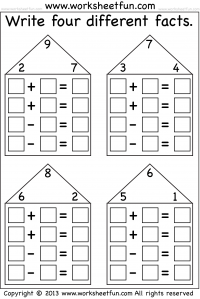Fact Family Houses – 7 Worksheets

Comment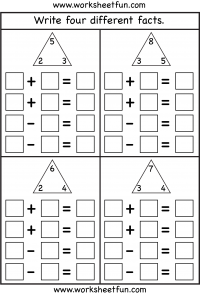Fact Family – Complete each fact family – 4 Worksheets

Comment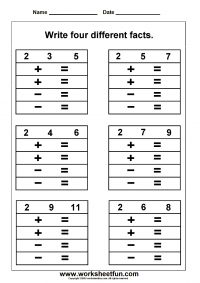Fact Family – Write four different facts – 2 Worksheets

Comment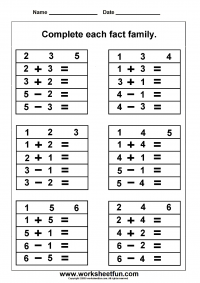Fact Family – Complete each fact family – 2 Worksheets

## Fact Families in First Grade Math

One of the more common concepts taught in first-grade math is the concept of a fact family or fact families.﻿﻿ Your child probably has first-grade math homework this year and may even ask you to help with it. If you've never heard of this, it's actually quite easy.

At this stage of development, your child is learning to do basic addition and subtraction. Fact families establish the relationships between numbers and aid in your child understanding the logic behind addition and subtraction.﻿﻿

Back to School in a Pandemic

### Helping With First Grade Math

A fact family is made up of three numbers. Just as in any family the members, or numbers, are related and there are always at least four math facts to be made with them. Take, for example, these members of a fact family: 6, 4, and 10.

### The Fact Family Relationships

In the family 6, 4, and 10 they are related because you can add two of the numbers to get the last number.

6 + 4 = 10

You can also switch the first two numbers, using the commutative property of addition, and still get the same answer.

4 + 6 = 10

### Fact Family Cousins

If addition is the direct relationship among these family members, then subtraction is the family cousin through the inverse property. Simply put, subtraction is the opposite of addition, but it's still related. The problems still only use the three members of the family.

10 - 4 = 6

10 - 6 = 4

### Keeping Track of All Family Members

Once your child knows the relationships of the fact family members, it's easy to see who is missing at a quick glance. Solving addition and subtraction problems is then much easier and starts to become automatic. Take, for example, this problem:

6 + ____ = 10

Your child should quickly be able to recognize 4 as the missing family member.

### Building a Fact Family House

A fun way to show the relationships of a fact family is to place the numbers in a house. Draw a typical house with a square box topped with a triangle for the attic and roof.

Now in the attic triangle, place the three numbers that will be part of the family in three attic windows. In this case, you'd place 4, 6, and 10 in the attic windows. In the main part of the house, place four large windows that contain the four different math operations of the fact family. These would be two for addition and two for subtraction:

__ + __ = __

__ - __ = __

Now your child can place the numbers appropriately in the equations and practice moving in their fact families. You can end up creating a whole neighborhood, such as each of the numbers that add up to 10.

This method of showing the relationships can make math a little more fun and approachable by your first-grader. Fact families help kids understand the patterns in mathematics.

Using Fact Family Houses

You can find other worksheets for fact families in many places online if you want different ones to use at home. Beyond addition and subtraction, you'll find multiplication and division fact families to use as your child advances to higher grades. There are apps and tablet games you can also find for fact family fun.

Tips to Help Children Succeed in First Grade

## Fact Family

When my son brought home his first Fact Family worksheet, I had no idea what I was looking at.

All I saw was a triangle with some numbers in it and a bunch of equations.

I'm not sure if this is a new way of teaching or if I've just forgotten about fact families.

Either way, we worked our way through it and handed in a perfect paper the next day.

He seems to work well with these types of problems so I thought I'd create a few math worksheets for him (and you) to supplement his homework.

### What Is A Fact Family?

While the numbered triangle confused me at first, the concept is simple.  The triangle contains three numbers that can all be used with each other to form 4 different equations.  There are addition and subtraction families along with multiplication and division families.  This page will deal with addition and subtraction facts.

Let's say we're given the following three numbers:  8, 6, and 2.  You can use these three numbers with addition and subtraction to arrive at the following equations:

6 + 2 = 8

2 + 6 = 8

8 - 6 = 2

8 - 2 = 6

The family reinforces basic addition facts and rules.  It shows that the order in which you add numbers together doesn't change the final result--this is also known as the Commutative Property of Addition.

These families also show the relationship and similarities between addition and subtraction.  For example, 10 - 8 = 2 is similar to 2 + 8 = 10.  Same numbers, different process.

When my son has trouble with subtraction (don't we all?) he has learned to turn it into an addition problem.  Using the example above, instead of trying to subtract 8 from 10 in his head, he figures out what he needs to add to 8 to get to 10.  I believe that studying these fact families has really improved his math skills.

### Fact Family Worksheets

Use the math worksheets below to build upon the concepts explained above.  There are two basic types of papers.

In the first set with the yellow triangles, the student is given the numbers and must then show the addition and subtraction relationships between them.

You'll notice a questions mark in some of the families.  Your child can write in any number to complete the problems.

The second set (featuring the school house) is a bit different.  Some of the numbers are already filled in, while others are missing.  Again, if there is a question mark, your child can enter any number.  One problem is missing all three numbers in the family.

Clicking a picture below will open a downloadable file in another tab.Solving Fact Family Houses: Addition and Subtraction

His chest, his eggs were burning with hellish fire, the rubber tissue did not give them freedom, his neck was like a scog. He was in charge of the female team and was happy, despite the more he had to endure. The head trainer of the "Cat" team, strong.

### You will also like:

The intercom call was not answered, but the door was opened. The clean and well-groomed entrance of a pretty brick high-rise building contrasted strongly with the filthy and painted entrances of typical panel houses, in which. I had more than once been on duty.

4356 4357 4358 4359 4360